# The Milky Way GalaxyPage 5

#### WATCH ALL SLIDES

Rmin = Ro sin l

ω(Rmin) = [vmax/(Ro sin l)] + ωo

By studying los longitude values from 0 to 90 degrees, Rmin will range from 0 to Ro

Limitations

No gas at subcentral point

Non-circular orbits

At Rmin = 0 and at Ro, difficult to measure curve due to small Doppler shifts

Measuring the Rotation Curve of the Milky Way

= Θo

= Θ

Slide 23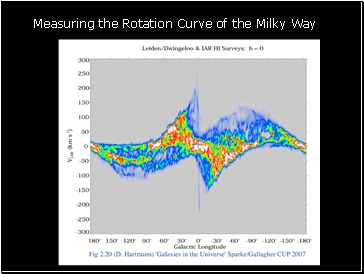Measuring the Rotation Curve of the Milky Way

Slide 24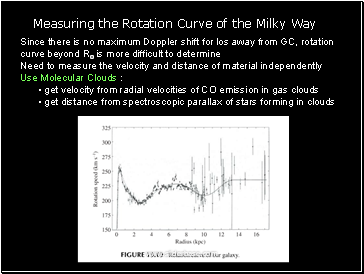Since there is no maximum Doppler shift for los away from GC, rotation curve beyond Ro is more difficult to determine

Need to measure the velocity and distance of material independently

Use Molecular Clouds :

get velocity from radial velocities of CO emission in gas clouds

get distance from spectroscopic parallax of stars forming in clouds

Measuring the Rotation Curve of the Milky Way

Slide 25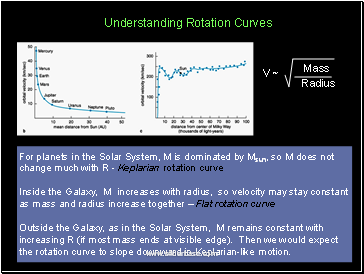For planets in the Solar System, M is dominated by Msun, so M does not change much with R - Keplarian rotation curve

Inside the Galaxy, M increases with radius, so velocity may stay constant as mass and radius increase together – Flat rotation curve

Outside the Galaxy, as in the Solar System, M remains constant with increasing R (if most mass ends at visible edge). Then we would expect the rotation curve to slope downward in Keplarian-like motion.

V ~

Understanding Rotation Curves

Slide 26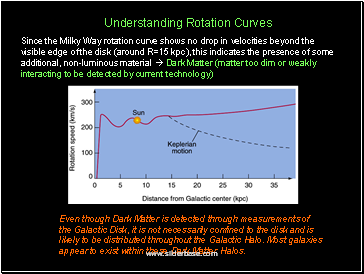Since the Milky Way rotation curve shows no drop in velocities beyond the visible edge of the disk (around R=15 kpc), this indicates the presence of some additional, non-luminous material  Dark Matter (matter too dim or weakly interacting to be detected by current technology)

Even though Dark Matter is detected through measurements of the Galactic Disk, it is not necessarily confined to the disk and is likely to be distributed throughout the Galactic Halo. Most galaxies appear to exist within these Dark Matter Halos.

Understanding Rotation Curves

Slide 27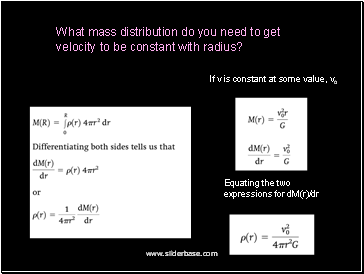If v is constant at some value, vo

Equating the two expressions for dM(r)/dr

What mass distribution do you need to get velocity to be constant with radius?

Slide 28## What is the Dark Matter?

Neutrinos: low mass particles that interact via gravity or weak nuclear force. Most neutrinos, produced from nuclear fusion, pass easily through the Sun. Common particles but low mass prevents them from contributing more than ~few percent of dark matter.

Go to page:
1  2  3  4  5  6  7  8